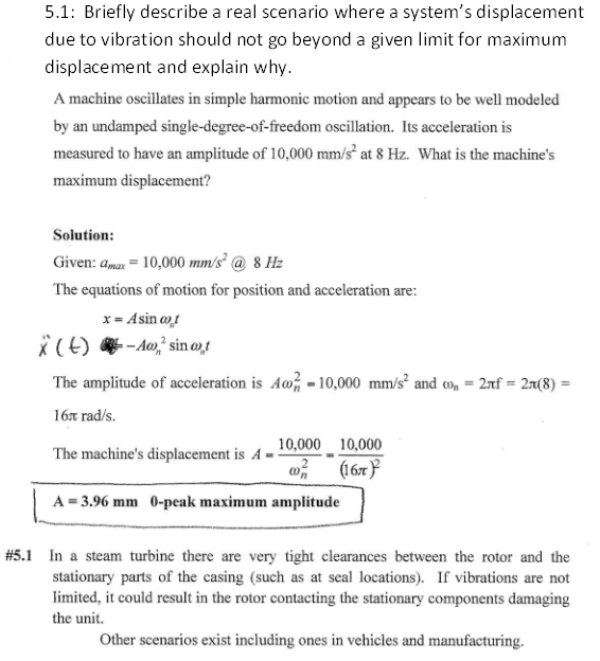A machine oscillates in simple harmonic motion and appears to be well modeled by an undamped single degree of freedom oscillation. Its acceleration is measured to have an amplitude of 10000 mm/s^2 at 8 Hz. What is the machine's maximum displacement? Briefly describe a real scenario where a system's displacement due to vibration should not go beyond a given limit for maximum displacement and explain why.A machine oscillates in simple harmonic motion and appears to be well modeled by an undamped single degree of freedom oscillation. Its acceleration is measured to have an amplitude of 10000 mm/s^2 at 8 Hz. What is the machine's maximum displacement? Briefly describe a real scenario where a system's displacement due to vibration should not go beyond a given limit for maximum displacement and explain why.

Vibrations Page 1 vibrations vibrations vibrations vibrations vibrations vibrations vibrations Vibrations Page 2